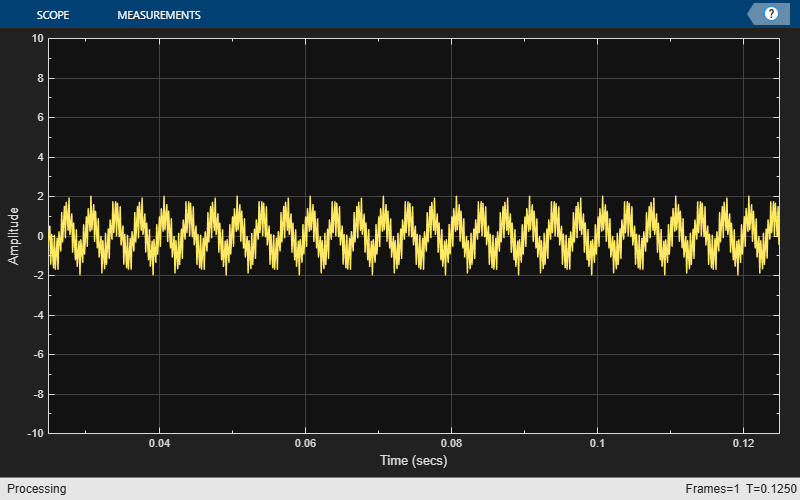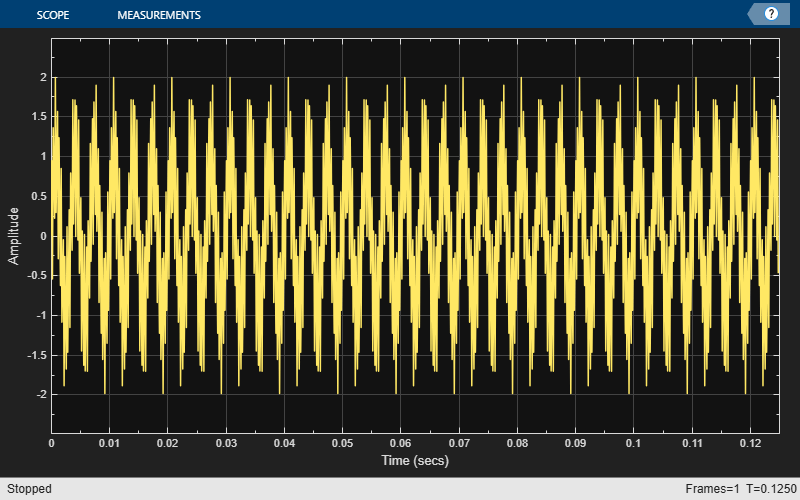# isVisible

Determine visibility of scope

Since R2020a

## Syntax

``visibility = isVisible(scope)``

## Description

````visibility = isVisible(scope)` returns the visibility of the scope as logical, with `1` (`true`) for visible.```

## Examples

collapse all

Create a sinusoidal signal with two tones, one at 0.3 kHz and the other at 3 kHz.

```t = (0:1000)'/8e3; xin = sin(2*pi*0.3e3*t)+sin(2*pi*3e3*t);```

Create a `timescope` object and view the sinusoidal signal by calling the time scope object `scope`.

```scope = timescope(SampleRate=8e3,... TimeSpanSource="property",... TimeSpan=0.1); scope(xin)```Run `release` to allow changes to property values and input characteristics. The scope automatically scales the axes.

`release(scope);`Hide the scope window.

```if(isVisible(scope)) hide(scope) end```

Show the scope window.

```if(~isVisible(scope)) show(scope) end```## Input Arguments

collapse all

Scope object whose visibility you want to query.

Example: ```myScope = timescope; visibility = isVisible(myScope)```

## Output Arguments

collapse all

If the scope window is open, the `isVisible` function returns `1` (`true`). Otherwise, the function returns `0` (`false`).

## Version History

Introduced in R2020a